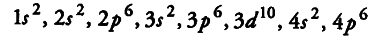# Give the name and atomic number of the inert gas atom

Give the name and atomic number of the inert gas atom in which the total number of d-electrons is equal to the difference in numbers of total p and s electrons.

The first inert gas which contains d electrons is krypton. Its atomic number is 36 and its electronic configuration isTotal number of ^-electrons = 10
Total number of /(-electrons = 6 + 6 + 6 = 18
Total number of .f-electrons = 2 + 2 + 2 + 2 = 8
.’. Difference in total number of p and s electrons = 18-8 = 10
Thus, the inert gas is krypton.